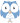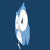Following on from the last page, we take the next step to build up the dimensional analysis query.

Continuing with f=ma as an example, and building on the exercise from Query 14.10, we repeat the analysis for all relevant dimensions (mass, length, time). If any of these fail to balance, then the formula fails. Finally, we invert this result, to find the formulas that pass the dimensional analysis test.

The queries in this section are based on the following data sets:

### Query ch14.10 Total exponents in each dimensionSince you did the exercise at the end of Query 14-10, this should come as no surprise. Using another VALUES definition, we iterate over all the dimensions we wish to analyze, and see the balance of the formula in each dimension. Already at this point, we see that the formula balaces.### Query ch14.11 Is there an unbalanced dimension?A formula fails if any of the dimensions fail to balance. We aggregate with GROUP BY ... SUM, then filter with HAVING, to find the formulas that fail; that is, any of the dimensions fails.### Query ch14.12 Verifying a formula with dimensional analysisTo pass dimensional analysis, we need to negate this result. We do this by putting a FILTER NOT EXISTS around the whole thing. This query returns TRUE if the formula passes, FALSE if it fails.

A few things to think about:

1. Why did we need to do the two steps, of checking ?formtotal!=0 (in Query 14.11), and then FILTER NOT EXISTS (in Query 14.12)? Couldn't we have just said ?formtotal=0 in the first place?
2. Think of another formula that you can check using this query. How would you edit the query to put in a new formula?